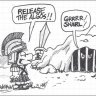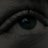# QQE (Quantitative Qualitative Estimation) for ThinkorSwim#### BenTen

Staff
VIP
QQE — or Quantitative Qualitative Estimation, is based on a rather complex calculation of the smoothed RSI indicators.

The QQE indicator consists of a smoothed Relative Strength Index (RSI) indicator and two volatility-based trailing levels (fast and slow). The Fast Trailing Level (TL) and Slow TL are constructed by calculating the ATR of the smoothed RSI over n-periods and then further smoothing the ATR using an additional n-periods Wilders smoothing function. This smoothed ATR of RSI is then multiplied by the Fast and Slow ATR Multipliers to calculate the final Fast and Slow Trailing Levels.### thinkScript Code

Code:
``````# QQE Indicator
# Converted by Kory Gill for BenTen at useThinkScript.com

declare lower;

input RSI_Period = 20;
input Slow_Factor = 5;
input QQE = 4.236;

def Wilder_Period = RSI_Period * 2 - 1;
def vClose = close;

def rsi = RSI(price = vClose, length = RSI_Period).RSI;
def rsi_ma = MovingAverage(AverageType.EXPONENTIAL, rsi, Slow_Factor);
def atr_rsi = AbsValue(rsi_ma - rsi_ma);
def atr_rsi_ma = MovingAverage(AverageType.EXPONENTIAL, atr_rsi, Wilder_Period);
def dar = MovingAverage(AverageType.EXPONENTIAL, atr_rsi_ma, Wilder_Period) * QQE;

def DeltaFastAtrRsi = dar;
def RSIndex = rsi_ma;
def newshortband =  RSIndex + DeltaFastAtrRsi;
def newlongband = RSIndex - DeltaFastAtrRsi;

def longband = if RSIndex > longband and RSIndex > longband
then max(longband,newlongband)
else newlongband;

def shortband = if RSIndex < shortband and  RSIndex < shortband
then min(shortband, newshortband)
else newshortband;

def trend = if Crosses(RSIndex, shortband)
then 1
else if Crosses(longband, RSIndex)
then -1
else if !IsNAN(trend)
then trend
else 1;

def FastAtrRsiTL = if trend == 1
then longband
else shortband;

plot pFastAtrRsiTL = FastAtrRsiTL;
plot pRsiMa = rsi_ma;
plot line50 = 50;

pFastAtrRsiTL.SetDefaultColor(CreateColor(225,109,47));
pRsiMa.SetDefaultColor(CreateColor(113,225,180));``````

••MerryDay, kcking, inhouselr and 9 others#### markos

##### Well-known member
VIP
Extremely accurate crossovers! Very Nice.

•BenTen
D

#### diazlaz

##### Well-known member
2019 Donor
VIP
per the information, in summary:

if the indicator band is much higher than the level of 50, there is uptrend;
if significantly below the level of 50, there is downward;
if the band fluctuates near level 50 (a little above or below the level), there is flat in the market.#### horserider

##### Well-known member
VIP
Same as other RSI indicators. Remember data can only be manipulated so much until results become redundant.
Thanks to diazlaz for again mentioning the importance of the 50 level of the RSI.

Suggest Ultimate RSI over this. Same signals essentially but more info in Ultimate RSI.

J

#### Jmp2626

##### New member
2019 Donor
@horserider You’re always spewing facts. Keep up the good work man. A lot of indicators are usually just transformations of others there’s only so many

••horserider and markos
S

#### skynetgen

##### Well-known member
A slightly modified version with easier to see signal https://tos.mx/TbwiboR
p.s. I wonder if there is a way to attach images to post without using imgurl. A picture is worth thousand words and imgurl process kinda convoluted

••MerryDay, inhouselr, TroyX and 1 other person
B

#### Barmarrs

##### New member
VIP
Not sure how I missed it before, but I like the simplicity of this one, too. Ultimate RSI is full of information, but it almost feels like information overload in one indicator - personal opinion.

I've tried toying around with QQE to create a scan, but I'm not having any luck. I have the scan set to pick up instances where RSI crosses above the Fast ATR, but nothing populates on the scan itself. Has anyone on here had any luck creating a scan for this one?

•Thomas#### BenTen

Staff
VIP
@Barmarrs You're correct about the scanner. I wasn't able to obtain any result either. I'll look more into this issue.

•Barmarrs
A

#### asragov

##### New member
2019 Donor
VIP
Thanks - pretty neat! - another question - what changes would need to be made to allow it work in an alert?

B

#### Barmarrs

##### New member
VIP
The issue seems to revolve around the Fast ATR - I can scan for signals where RSI crosses above the 50 line but nothing else.

Does anyone know if it'd be possible to turn this indicator into a strategy? My knowledge with ThinkScript and writing code is pretty much non-existent, but if someone has any tips/hints or can share a starting place, I can toy around with it.#### BenTen

Staff
VIP
@Barmarrs Define your condition for buying and selling then we will put together a backtesting strategy for you.

B

#### Barmarrs

##### New member
VIP
@BenTen Buying condition would be where the RSI plot crosses the Fast ATR; selling would be the Fast ATR crossing the RSI plot.

Not sure if it's possible with the issue I've noticed with the scanner, but I wanted to check. Thanks for the reply and again for putting this forum together! I can't speak for everyone, but the indicators, resources, and collaboration from others I've seen have really helped me develop new skills and fine tune others - it's really appreciated!

Sorry for the unending questions on this one, but is there a way to add up/down arrows on the candles themselves when the the RSI crosses the ATR line? Specifically an up arrow where the RSI crosses above the ATR, and a down arrow when the ATR crosses the RSI? I'm striking out on all fronts in trying to get a working scanner/strategy for this one, but I'm loving the signals I've seen on AMM 2.0 combined with this indicator. I've never used ToS support, and I'm not sure what their limitations are, but would they go through the code to assist with questions like this?

If it can't be done, I understand - I just wanted to make sure. It should go without saying, but thanks again for putting the time and effort in to this forum!

Last edited by a moderator:
•BenTen#### BenTen

Staff
VIP
@Barmarrs Add this to the bottom of the script:

Code:
``````input over_sold = 20;
input over_bought = 80;

plot OverSold = over_Sold;
plot OverBought = over_Bought;
plot UpSignal = if pRSIMA crosses above pFastAtrRsiTL then OverSold else Double.NaN;
plot DownSignal = if pRSIMA crosses below pFastAtrRsiTL then OverBought else Double.NaN;

UpSignal.SetDefaultColor(Color.UPTICK);
UpSignal.SetPaintingStrategy(PaintingStrategy.ARROW_UP);
DownSignal.SetDefaultColor(Color.DOWNTICK);
DownSignal.SetPaintingStrategy(PaintingStrategy.ARROW_DOWN);``````

••TroyX and Barmarrs
K

#### kelvin

##### Member
I saw this indicator somewhere else, it looks great with K bars and Histogram could show you very clear entry and exit points.

Code:
``````{ Quantitative Qualitative Estimation Indicator Revised}

inputs:
double RSIPrice(                        Close )[DisplayName = "RSIPrice", ToolTip =
"Price to be used in the calculation of the RSI."],
int RSILength(                        14 )[DisplayName = "RSILength", ToolTip =
"The number of bars to be used in the calculation of the RSI."],
int RSISmoothLength(                        5 )[DisplayName = "RSISmoothLength", ToolTip =
"The length of the exponential moving average of the RSI.  This value determines the smoothing factor used in the exponential moving average calculation."],
int ATRLength(                        27 )[DisplayName = "ATRLength", ToolTip =
"The length of the exponential moving average of the true range of the smoothed RSI."],
int ATRSmoothLength(                        27 )[DisplayName = "ATRSmoothLength", ToolTip =
"The length of the exponential moving average of the moving average of the true range of the smoothed RSI."],
double FastATRMult(                        2.618 )[DisplayName = "FastATRMult", ToolTip =
"Fast trendline scaling factor that is multiplied by the moving average of the moving average of the true range of the smooth RSI."],
double SlowATRMult(                        4.236 )[DisplayName = "SlowATRMult", ToolTip =
"Slow trendline scaling factor that is multiplied by the moving average of the moving average of the true range of the smooth RSI."],
"If alerts are enabled for the indicator, an alert will be triggered if the smoothed RSI crosses the level specified by this input"],

LRSLength(3),
Multiple(2.38),

OverBought1(60),
OverBought2(70),
OverSold1(40),
OverSold2(30),

HUpColor(green),
HDwnColor(magenta),
LUpColor(Cyan),
LDwnColor(Red),
MUpColor(Green),
MDwnColor(Red),
BarUpColor(green),
BarDwColor(red),

variables:
double RetVal( 0 ),
double oSmoothRSI( 0 ),
double oFastTL( 50 ),
double oSlowTL( 50 ),
intrabarpersist bool OkToPlot( false ),
LRS(0);

RetVal = QQE( RSIPrice, RSILength, RSISmoothLength, ATRLength, ATRSmoothLength,
FastATRMult, SlowATRMult, oSmoothRSI, oFastTL, oSlowTL );

Value1 = Highest(oSmoothRSI, 2);

Value2 = Lowest(oSmoothRSI, 2);

if oSmoothRSI > oSmoothRSI then

Value3 = Value1 - Value2 ;

if oSmoothRSI < oSmoothRSI Then

Value3 = Value2 - Value1 ;

LRS = LinearRegSlope(oSmoothRSI, LRSLength);

{ do not plot until all exponential moving averages have stabilized }
once ( CurrentBar > 4 * MaxList( RSILength, RSISmoothLength, ATRLength,
ATRSmoothLength ) )
begin
OkToPlot = true;
end;

if OkToPlot then
begin
Plot1( oSmoothRSI, !( "SmoothRSI" ) );
Plot2( oFastTL, !( "FastTL" ) );
Plot3( oSlowTL, !( "SlowTL" ) );
Plot4( SmoothRSIAlertLevel, !( "SmRSIAlrtLev" ) );
Plot5(OverBought1, "OverBought1");
Plot6(OverSold1, "OverSold1");
Plot7(Value1, "BH");
Plot8(Value2, "BL");
Plot9(OverBought2, "OverBought2");
Plot10(OverSold2, "OverSold2");
Plot12(LRS * Multiple, "LRS-SRSI");

var: var1(yellow), var4(yellow), var7(yellow), var8(yellow), var11(yellow);

If currentbar >= 0 then begin

If oSmoothRSI >= SmoothRSIAlertLevel then begin
if oSmoothRSI > oFastTL then var1 = HUpColor;
if oSmoothRSI < oFastTL then var1 = HDwnColor;
end;

If oSmoothRSI < SmoothRSIAlertLevel then begin
if oSmoothRSI > oFastTL then var1 = LUpColor;
if oSmoothRSI < oFastTL then var1 = LDwnColor;
end;

SetPlotColor(1,var1);

If oSmoothRSI >= SmoothRSIAlertLevel then var4 = MUpColor;

If oSmoothRSI < SmoothRSIAlertLevel then var4 = MDwnColor;

SetPlotColor(4,var4);

if oSmoothRSI > oSmoothRSI then var7 = BarUpColor;

if oSmoothRSI > oSmoothRSI then var8 = BarUpColor;

if oSmoothRSI < oSmoothRSI then var7 = BarDwColor;

if oSmoothRSI < oSmoothRSI then var8 = BarDwColor;

SetPlotColor(7,var7);

SetPlotColor(8,var8);

If Value3 >= 0  Then var11 = SpreadUpColor;

If Value3 < 0 Then var11 = SpreadDnColor;

SetPlotColor(11,var11);

End;

begin
if oSmoothRSI crosses over SmoothRSIAlertLevel then
begin
Alert( !( "SmoothRSI crossed over" ) + " " +
end
else if oSmoothRSI crosses under SmoothRSIAlertLevel then
begin
Alert( !( "SmoothRSI crossed under" ) + " " +
end;
end;
end;``````

it comes with over bought and over sold level.

This revised version comes with 3 lines cross over, over bought/over sold levels, K bars in the main signal line can change colors when trends reversed, also attached a histogram, very fancy, should be best and popular indicator in TradeStation

Last edited:
•#### BenTen

Staff
VIP
@kelvin What does the one on TradeStation looks like? It sounds like you just want some cosmetic stuff (such as overbought and oversold area) added.

K

#### kelvin

##### Member•#### BenTen

Staff
VIP
@kelvin Add this snippet at the bottom of the QQE indicator I posted in the Warehouse forum and you will get the same overbought and oversold levels.

Code:
``````plot ob1 = 70;
plot ob2 = 60;
plot os1 = 40;
plot os2 = 30;``````

•kelvin
T

##### Active member

•BenTen
T

##### Active member
2019 Donor
@kelvin This above code has errors in it has it been revised ??

T

#### Thomas

##### Active member
VIP
Syracuse is am amazing designer,...you can also find him on Twitter,.....https://tos.mx/QtrcCwwQQE MTF (Multi Timeframe) for ThinkorSwim Indicators 11